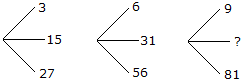# Verbal Reasoning - Character Puzzles - Discussion

### Discussion :: Character Puzzles - Character Puzzles 1 (Q.No.5)

5.

Which one will replace the question mark ?[A]. 45 [B]. 41 [C]. 32 [D]. 40

Explanation:

(15 x 2 - 3) = 27,

(31 x 2 - 6) = 56

and (45 x 2 - 9) = 81

 Kiran said: (Oct 29, 2010) step-1 27+3=30/2=15. step-2 56+6=62/2=31. step-3 9+81=90/2=45

 Ambika said: (Dec 27, 2010) Thanks Kiran.

 Suresh said: (Jan 29, 2011) step 1 : 1*3=3,5*3=15,9*3=27 step 2 : 1*6+0=6,5*6+1=31,9*6+2=56 step 3 : 1*9=9,5*9=45,9*9=81

 Poornima said: (Oct 24, 2011) (27+3)/2=15 (6+56)/2=31 (81+9)/2=45

 Muralikrishna Maddu said: (Feb 5, 2014) 3+12 = 15+12 = 27 -- -3,15,27. 6+25 =31+25 = 56 --- 6,31,56. 9+36 = 45+36 = 81 --- 9,45,81.

 Neeraj Singh Gaira said: (Aug 2, 2014) Logic is mid = (up+end)/2. (3+27)/2 = 15. (6+56)/2 = 31. So (9+81)/2 = 45.

 Pawan Yadav said: (Sep 5, 2015) (27+3) = 15. (6+56) = 31. Then (81+9) = ?

 Ajith said: (Sep 7, 2017) Awesome, Thanks @Kiran.

 Nishant said: (Jul 6, 2018) (27+3)/2 = 15. (56+6)/2 = 31. (81+9)/2 = 45.

 Abid Mohammad said: (Sep 4, 2018) (15*2) -3=27. (31*2) -6=56 //gap between these numbers (15 to 31) is 16. (45*2) -9=81 //gap between these numbers (31 to 14) is 14.

 Tej Bahadur Budhathoki said: (Oct 16, 2019) 15-3 = 12+15 = 27. 31-6 = 25+31 = 56. 45-9 = 36+45 = 81.

 Kapil P said: (Jan 11, 2020) (27+3)÷2 = 15, (56+6)÷2 = 31, (81+9)÷2 = 45.

 Raj said: (Oct 29, 2020) 27+3 = 30, 30÷2 = 15. 56+6 = 62, 62÷2 = 31. 81+9 = 90, 90÷2 = 45.

 Sughi said: (Nov 14, 2020) 27+3÷2 = 15. 56+6÷2 = 31. 81+9÷2 = 45.

 Pavankumar said: (Aug 14, 2021) 3*3 = 9, 15*3 = 45, 27*3 = 81.